Question

Two blocks of masses m1 = 1.82 kg and m2 = 4.17 kg are each released...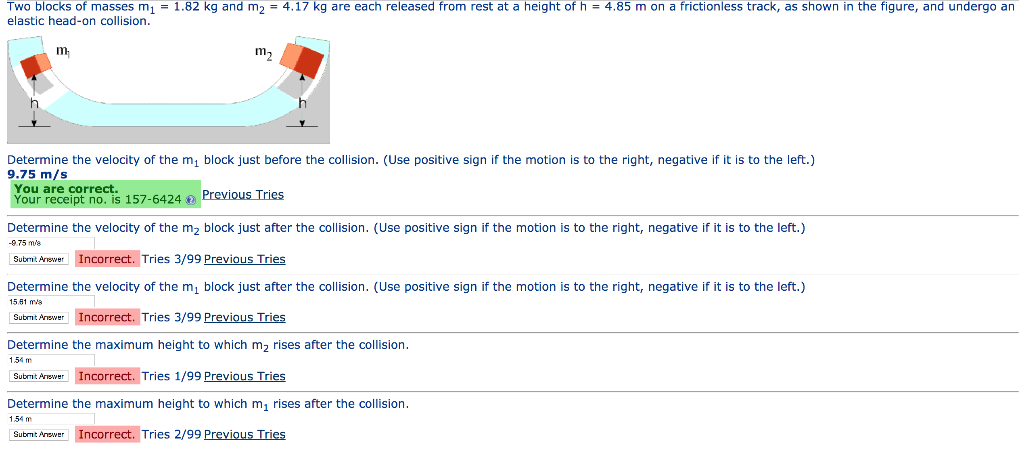Two blocks of masses m1 = 1.82 kg and m2 = 4.17 kg are each released from rest at a height of h = 4.85 m on a frictionless track, as shown in the figure, and undergo an elastic head-on collision m m2 Determine the velocity of the mi block just before the collision. (Use positive sign if the motion is to the right, negative if it is to the left.) 9.75 m/s You are correct. Your receipt no. is 157-6424 Previous Tries Determine the velocity of the m2 block just after the collision. (Use positive sign -975 m the motion is to the right, negative if it is to the left.) Incorrect. Tries 3/99 Previous Tries Submt Anewer Determine the velocity of the mi block just after the collision. (Use positive sign the motion is to the right, negative if it is to the left.) 15.81 m Incorrect. Tries 3/99 Previous Tries Submt Answer Determine the maximum height to which m2 rises after the collision 154 m Sutmit Ariewer Incorrect. Triess 1/99 Previous Tries Determine the maximum height to which m, rises after the collision 154 m Incorrect. Tries 2/99 Previous Tries Submt Anewer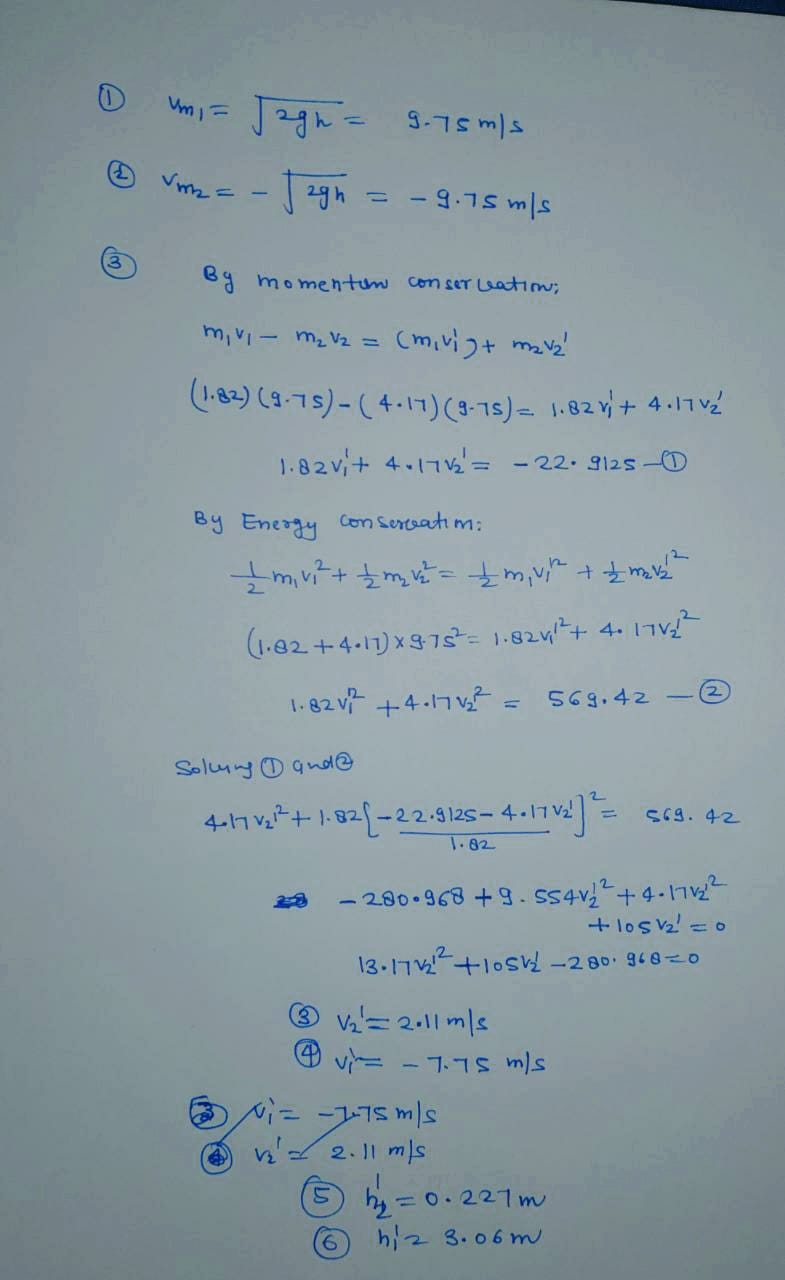Earn Coins

Coins can be redeemed for fabulous gifts.

Similar Homework Help Questions
• Two blocks of masses m1=2.00 kg and m2=4.00 kg are each released from..

Two blocks of masses m1=2.00kg and m2=4.00 kg are each released from rest at a height y=4.40 m on a frictionless track and undergo an elastic head-on-collision. Whatis the maximum height to which m1 rises after the collision?-Please explain step by step, im so confused :(

• Figure shown above.Please show all work with algebra. На m2 Problem 3. 3. Two blocks of...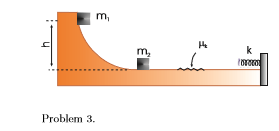Figure shown above.Please show all work with algebra. На m2 Problem 3. 3. Two blocks of masses m-1.0 kg and m2 2.0 kg are free to slide along a wooden track with a spring of force constant k 1000 N/m at one end, as shown in the figure below. The wooden track is frictionless except for a length of 1.5 m, where the coefficient of kinetic friction is 0.25. The block mi is released from rest at a height 1.0...

• As shown in the figure below, two blocks (m1 and m2) are each released from rest...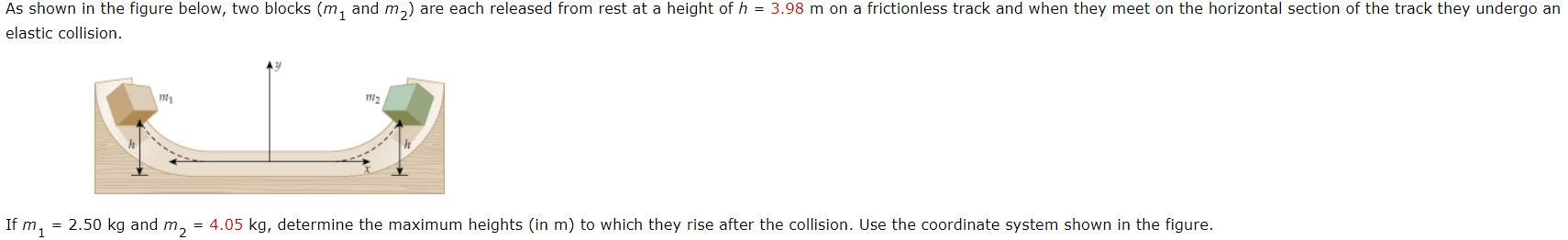As shown in the figure below, two blocks (m1 and m2) are each released from rest at a height of h = 3.98 m on a frictionless track and when they meet on the horizontal section of the track they undergo an elastic collision. If m1 = 2.50 kg and m2 = 4.05 kg, determine the maximum heights (in m) to which they rise after the collision. Use the coordinate system shown in the figure. As shown in the figure...

• As shown in the figure below, two blocks (m1 and m2) are each released from rest...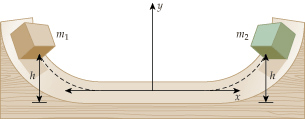As shown in the figure below, two blocks (m1 and m2) are each released from rest at a height of h = 3.83 m on a frictionless track and when they meet on the horizontal section of the track they undergo an elastic collision. If m1 = 2.50 kg and m2 = 4.45 kg,determine the maximum heights (in m) to which they rise after the collision. Use the coordinate system shown in the figure. y1f = m y2f = m...

• As shown in the figure below, two blocks (m1 and m2) are each released from rest...As shown in the figure below, two blocks (m1 and m2) are each released from rest at a height of h = 3.28 m on a frictionless track and when they meet on the horizontal section of the track they undergo an elastic collision. If m1 = 2.50 kg and m2 = 4.75 kg, determine the maximum heights (in m) to which they rise after the collision. Use the coordinate system shown in the figure. y1f = m y2f =...

• Two blocks of masses m1 2.00 kg and m2 4.55 kg are released from rest at...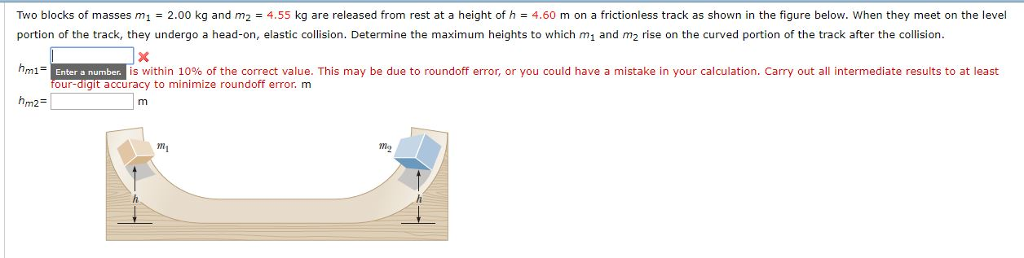Two blocks of masses m1 2.00 kg and m2 4.55 kg are released from rest at a height of h 4.60 m on a frictionless track as shown in the figure below. When they meet on the level portion of the track, they undergo a head-on, elastic collision. Determine the maximum heights to which m1 and m2 rise on the curved portion of the track after the collision hm1 is within 10% of the correct value. This may be due...

• Two particles of mass m1 = 2.0 kg and m2 = 2.6 kg undergo a one-dimensional...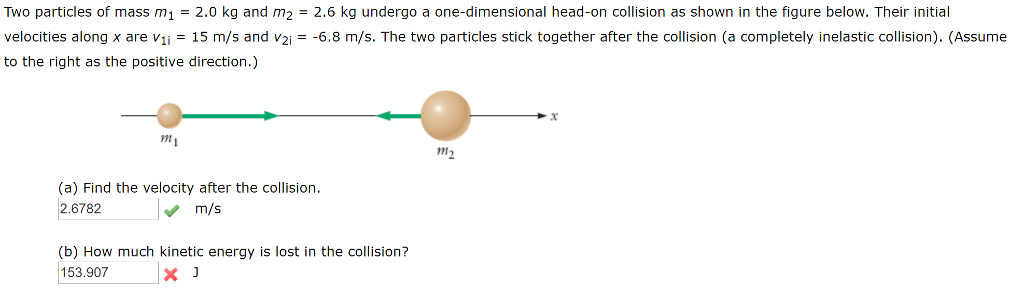Two particles of mass m1 = 2.0 kg and m2 = 2.6 kg undergo a one-dimensional head-on collision as shown in the figure below. Their initial velocities along x are vii = 15 m/s and v2,--6.8 m/s. The two particles stick together after the collision (a completely inelastic collision. (Assume to the right as the positive direction.) mi m2 (a) Find the velocity after the collision. 2.6782 m/s (b) How much kinetic energy is lost in the collision? 153.907x

• As shown in the figure below, two blocks m1 and m2 are each released from rest...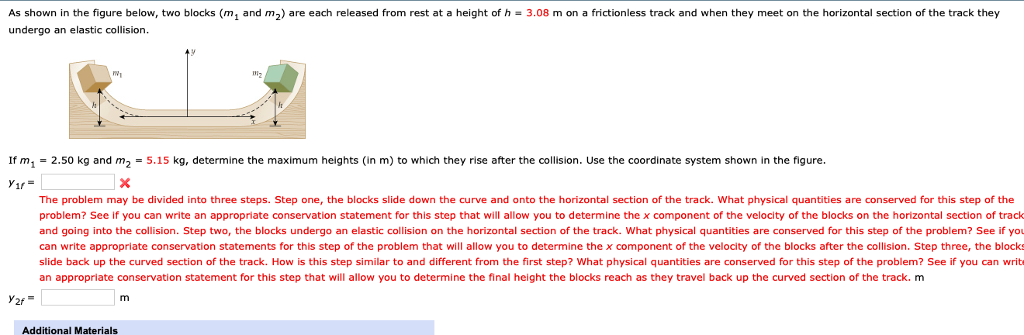As shown in the figure below, two blocks m1 and m2 are each released from rest at a height of h·3.08 m on a frictionless track and when they meet on the horizontal section of the track they undergo an elastic collision. If m1 = 2.50 kg and m2 = 5.15 kg, determine the maximum heights (in m) to which they rise after the collision. Use the coordinate system shown in the figure. Yir The problem may be divided into...

• Two blocks have masses mi = 5 kg and m2 = 1 kg and speeds v1...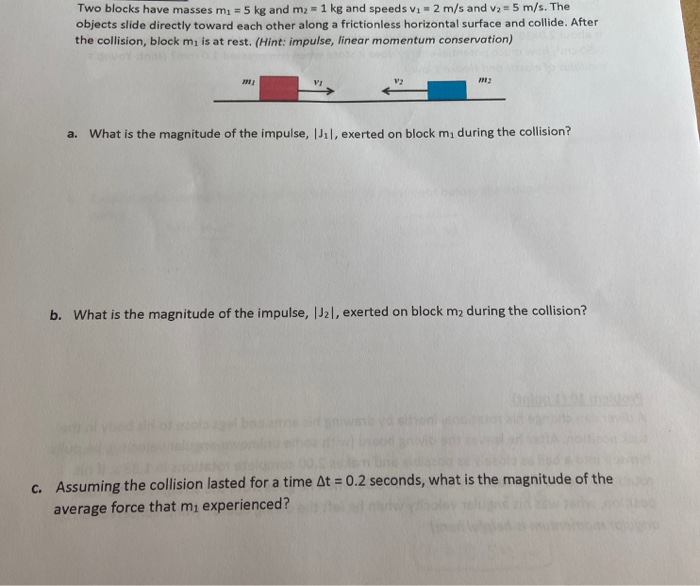Two blocks have masses mi = 5 kg and m2 = 1 kg and speeds v1 = 2 m/s and v2 = 5 m/s. The objects slide directly toward each other along a frictionless horizontal surface and collide. After the collision, block mi is at rest. (Hint: impulse, linear momentum conservation) a. What is the magnitude of the impulse, Jil, exerted on block mı during the collision? b. What is the magnitude of the impulse, 1J21, exerted on block me...

• As shown in the figure below, two blocks (m1 and m2) are each released from rest...

As shown in the figure below, two blocks (m1 and m2) are each released from rest at a height of  h = 4.18 m on a frictionless track and when they meet on the horizontal section of the track they undergo an elastic collision. If m1 = 2.50 kg and m2 = 4.15 kg,determine the maximum heights (in m) to which they rise after the collision. Use the coordinate system shown in the figure. y1f= ??m y2f=????m